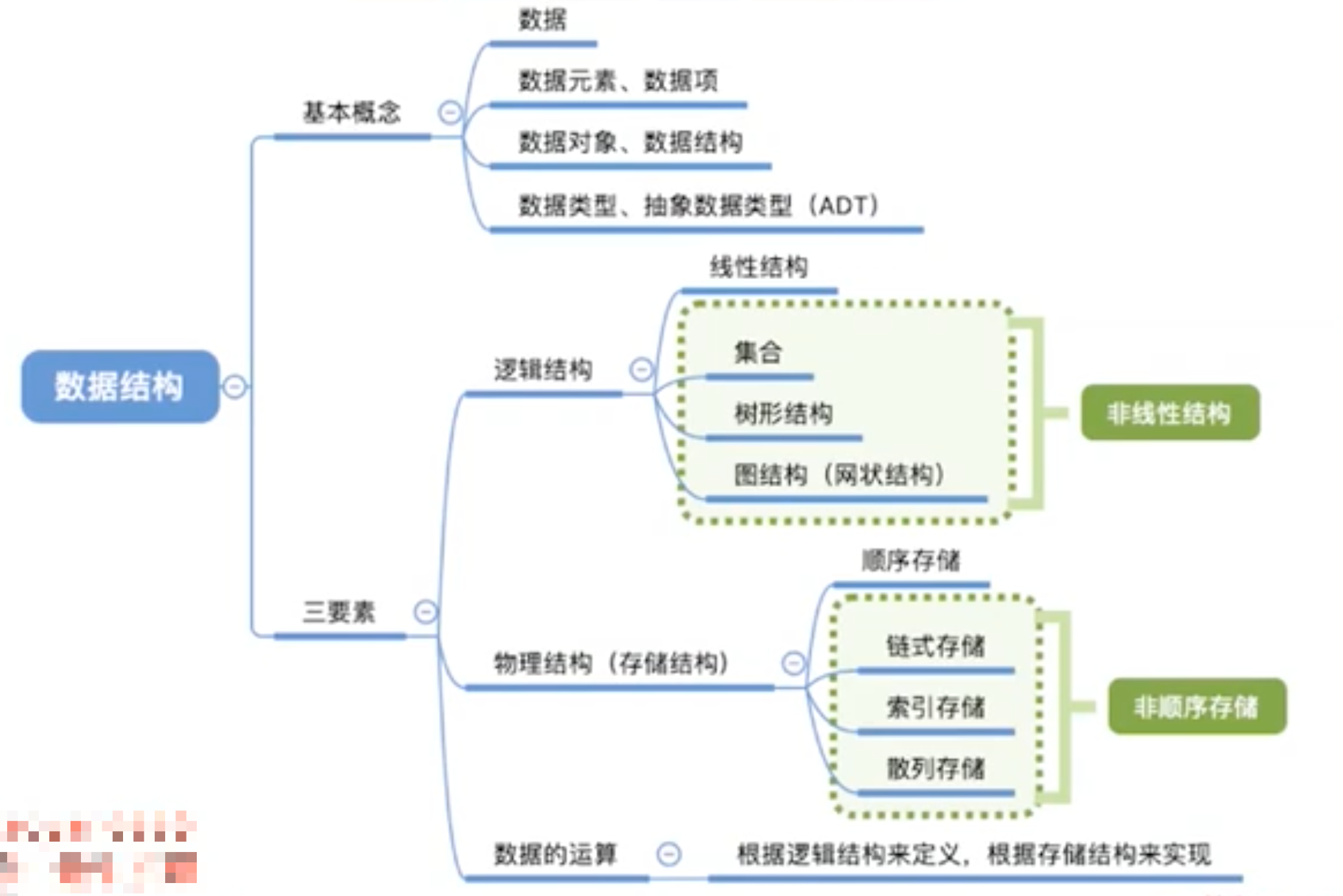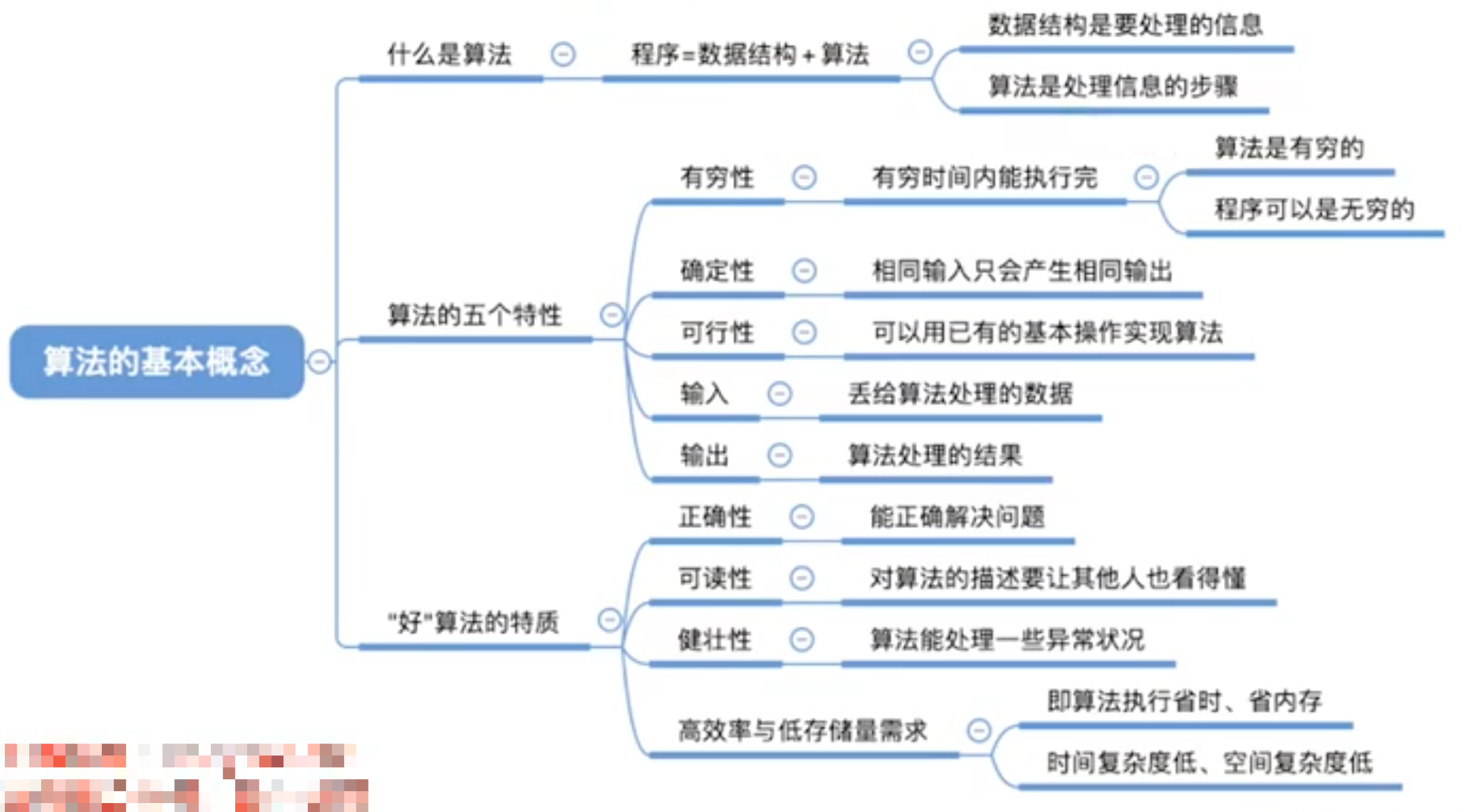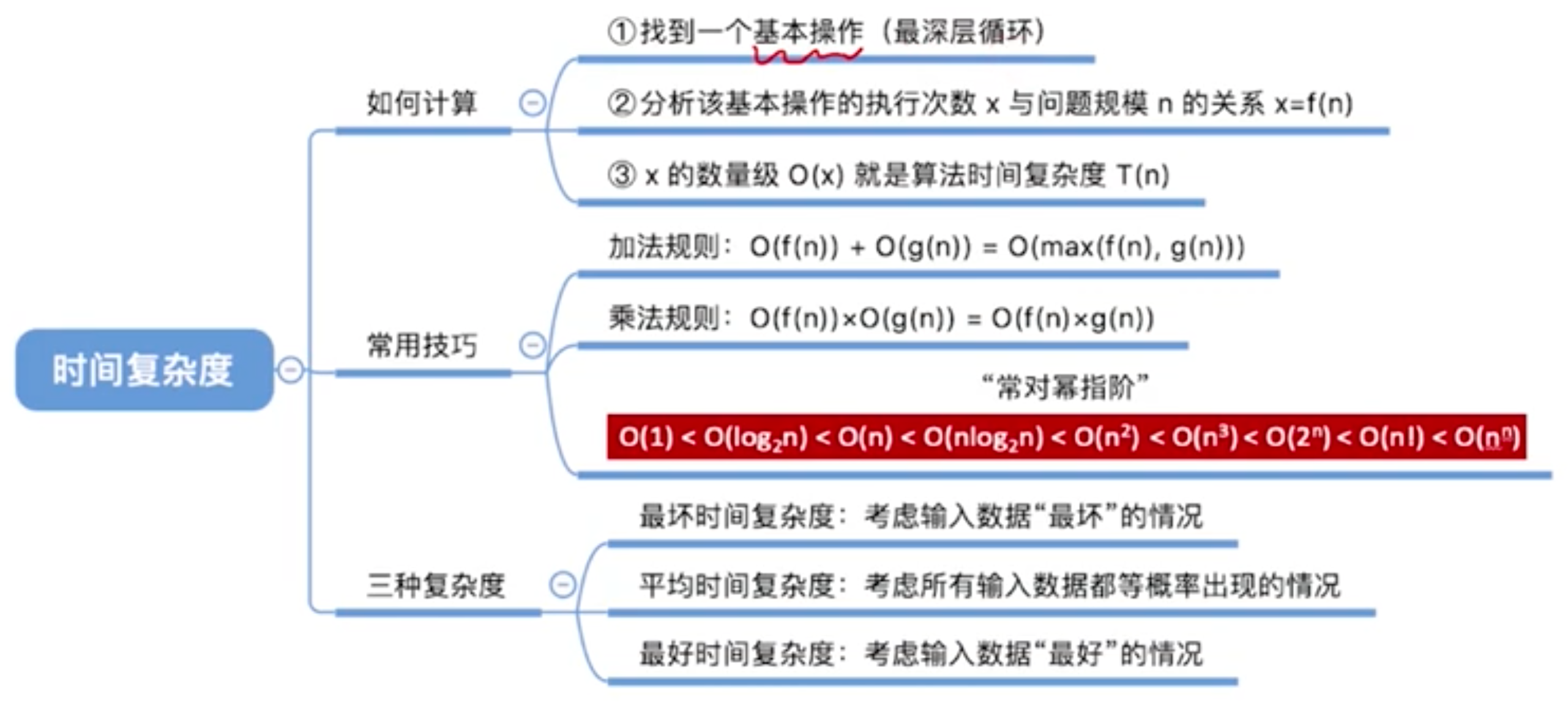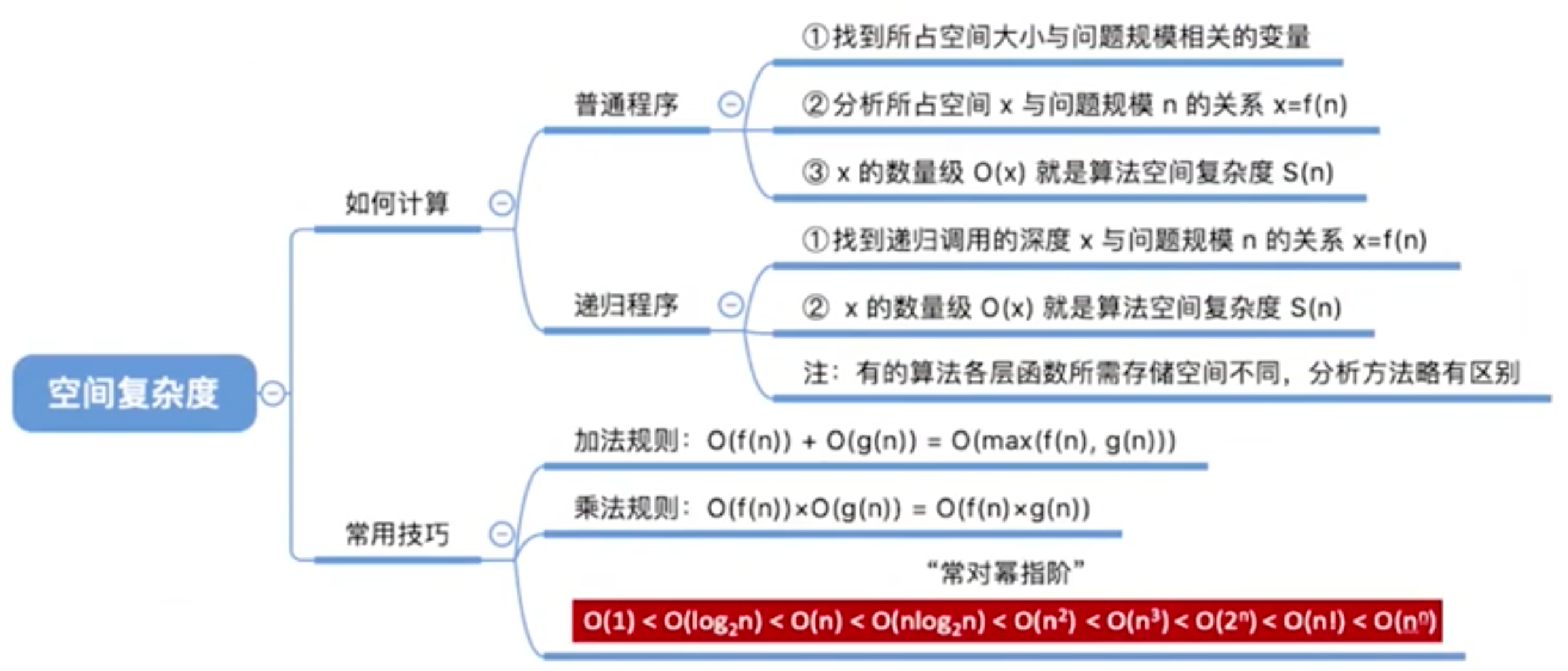# 数据结构基本概念## 数据结构的三要素

• 逻辑结构 - 数据元素之间的逻辑关系
• 集合
• 各个元素同属于一个集合，别无其他关系
• 线性结构
• 数据元素之间是一对一的关系除了第一个元素
• 除了第一个元素，所有元素都有唯一前驱
• 除了最后一个元素，所有元素都有唯一后继
• 树形结构
• 数据元素之间是一对多的关系
• 图状结构
• 数据元素之间是多对多的关系
• 物理结构（存储结构）
• 顺序存储：把逻辑上相邻的元素存储在物理位置上也相邻地存储单元中，元素之间的关系由存储单元的邻接关系来体现。
• 链式存储：逻辑上相邻的元素在物理位置上可以不相邻，借助指示元素存储地址的指针来表示元素之间的逻辑关系。
• 索引存储：在存储元素信息的同时，还简历附加的索引表。索引表中的每项称为索引项，索引项的一般形式是（关键字，地址）。
• 散列存储：根据元素的关键字直接计算出该元素的存储地址，又称哈希 Hash 存储。
• 数据的运算 - 施加在数据上的运算包括运算的定义和实现
• 运算的定义是针对逻辑结构的，指出运算的功能。
• 运算的实现是针对存储结构的，指出运算的具体操作步骤。

### 重点

1. 若采用顺序存储，则各个数据元素在物理上必须是连续的；若采用非顺序存储，则各个数据元素在物理上可以是离散的
2. 数据的存储结构会影响存储空间分配的方便程度
3. 数据的存储结构会影响对数据运算的速度

## 数据类型、抽象数据类型

1. 原子类型：其值不可再分的数据类型
2. 结构类型：其值可以再分解为若干成分（分量）的数据类型

## 探讨一种数据结构的步骤

1. 定义逻辑结构（逻辑元素之间的关系）
2. 定义数据的运算（针对现实需求，应该对这种逻辑结构进行什么用的运算）
3. 确定某个存储结构，实现数据结构，并实现一些对数据结构的基本运算

# 算法基本概念## 算法的特性

• 有穷性：一个算法必须在执行有穷步之后结束，且每一步都可在有穷时间内完成
• 确定性：算法中每条指令必须有确切的含义，对于相同的输入只能得出相同的输出。
• 可行性：算法中描述的操作都可以通过已经实现的基本运算执行有限次来实现。
• 输入：一个算法有零个或多个输入，这些输入取自于某个特定的对象的集合。
• 输出：一个算法有一个或多个输出，这些输出是与输入有着某种特定关系的量。

## 「好」算法的特质

• 正确性：算法应能够正确地解决求解问题。
• 可读性：算法应具有良好的可读性，以帮助人们理解。
• 健壮性：输入非法数据时，算法能适当地做出反应或进行处理，而不会产生莫名其妙的输出结果。
• 效率与存储量：效率是指算法执行时间，存储量需求是指算法执行过程中所需最大存储空间。

# 算法效率的度量

## 复杂度的大 O 符号

O(1) < O(log2n) < O(n) < O(n*log2 n) < O(n2) < O(n3) < O(2n) < O(n!) < O(nn)

## 评估算法时间复杂度### 存在什么问题

• 和机器性能有关
• 和编译语言有关，越高级的语言执行效率越低
• 和编译程序产生的机器指令质量有关
• 有些算法是不能事后再统计的

## 算法的时间复杂度

• 最坏时间复杂度：最坏情况下算法的时间复杂度
• 平均时间复杂度：所有输入示例相等概率出现的情况下，算法的期望运行时间
• 最好时间复杂度：最好情况下算法的时间复杂度

• T1(n) = 3n + 3 ≈ 3n
• T2(n) = n2 + 3n + 3 ≈ n2
• T3(n) = n3 + n2 + 9999 ≈ n3

### 结论

• 可以只考虑阶数高的部分
• 多项相加，只保留最高阶的项，且系数变为 1
• T(n) = T1(n) + T2(n) = O(f(n)) + O(g(n)) = O(max(f(n), g(n)))
• 多项相乘，都保留
• T(n) = T1(n) * T2(n) = O(f(n)) * O(g(n)) = O(f(n) * g(n))
• 顺序执行的代码只会影响常数项，可以忽略
• 只需选择循环中的一个基本操作分析它的执行次数与 n 的关系即可
• 如果有多层嵌套循环，只需关注最深层循环循环了几次

## 评估算法空间复杂度## 算法的空间复杂度

### 普通程序

• 找到所占空间大小与问题规模相关的变量
• 分析所占空间 x 与问题规模 n 的关系 x = f(n)
• x 的数量级 O(x) 就是算法空间复杂度 S(n)

### 递归程序

• 找到递归调用的深度 x 与问题规模 n 的关系 x = f(n)
• x 的数量级 O(x) 就是算法空间复杂度 S(n)
• 有的算法各层函数所需存储空间不同，分析方法略有区别

This is the end of post